# What are the different independence assumptions in hMM & Naive Bayes ?

Both the hMM and Naive Bayes have conditional independence assumption.

hMM can be expressed by the equation below :Second equation implies a conditional independence assumption: Given the state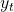observed variable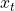is conditionally independent of previous observed variables, i.e.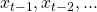and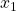Naive Bayes Model is expressed as: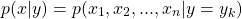is the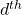feature for the data sample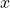and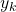is thelabel forclass problem.

The above equation can be written asThis implies a conditional independence assumption: given the class label, data features are independent of each other.